# The Complete Guide to Understanding Control Charts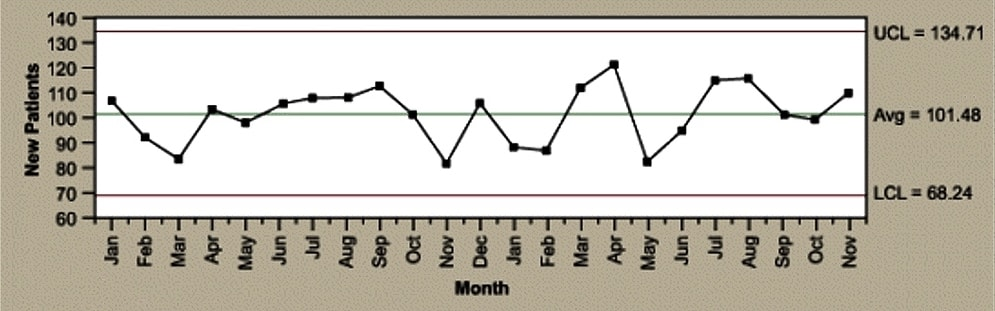By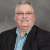Carl Berardinelli

Control charts have two general uses in an improvement project.

The most common application is as a tool to monitor process stability and control.

A less common, although some might argue more powerful, use of control charts is as an analysis tool.

The descriptions below provide an overview of the different types of control charts to help practitioners identify the best chart for any monitoring situation, followed by a description of the method for using control charts for analysis.

### Identifying Variation

When a process is stable and in control, it displays common cause variation, variation that is inherent to the process. A process is in control when based on past experience it can be predicted how the process will vary (within limits) in the future. If the process is unstable, the process displays special cause variation, non-random variation from external factors.

Control charts are simple, robust tools for understanding process variability.

### The Four Process States

Processes fall into one of four states: 1) the ideal, 2) the threshold, 3) the brink of chaos and 4) the state of chaos (Figure 1).3

When a process operates in the ideal state, that process is in statistical control and produces 100 percent conformance. This process has proven stability and target performance over time. This process is predictable and its output meets customer expectations.

A process that is in the threshold state is characterized by being in statistical control but still producing the occasional nonconformance. This type of process will produce a constant level of nonconformances and exhibits low capability. Although predictable, this process does not consistently meet customer needs.

The brink of chaos state reflects a process that is not in statistical control, but also is not producing defects. In other words, the process is unpredictable, but the outputs of the process still meet customer requirements. The lack of defects leads to a false sense of security, however, as such a process can produce nonconformances at any moment. It is only a matter of time.

The fourth process state is the state of chaos. Here, the process is not in statistical control and produces unpredictable levels of nonconformance.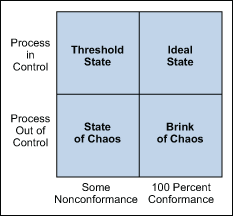Figure 1: Four Process States

Every process falls into one of these states at any given time, but will not remain in that state. All processes will migrate toward the state of chaos. Companies typically begin some type of improvement effort when a process reaches the state of chaos (although arguably they would be better served to initiate improvement plans at the brink of chaos or threshold state). Control charts are robust and effective tools to use as part of the strategy used to detect this natural process degradation (Figure 2).3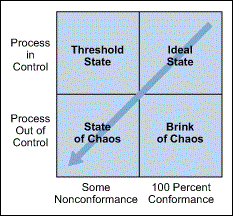### Elements of a Control Chart

There are three main elements of a control chart as shown in Figure 3.

1. A control chart begins with a time series graph.
2. A central line (X) is added as a visual reference for detecting shifts or trends – this is also referred to as the process location.
3. Upper and lower control limits (UCL and LCL) are computed from available data and placed equidistant from the central line. This is also referred to as process dispersion.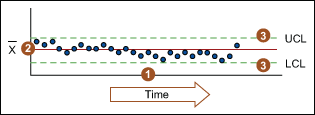Figure 3: Elements of a Control Chart

Control limits (CLs) ensure time is not wasted looking for unnecessary trouble – the goal of any process improvement practitioner should be to only take action when warranted. Control limits are calculated by:

1. Estimating the standard deviation, σ, of the sample data
2. Multiplying that number by three
3. Adding (3 x σ to the average) for the UCL and subtracting (3 x σ from the average) for the LCL
Handpicked Content:   Integrating SPC and SQC to Overcome Weaknesses in Each

Mathematically, the calculation of control limits looks like: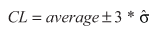(Note: The hat over the sigma symbol indicates that this is an estimate of standard deviation, not the true population standard deviation.)

Because control limits are calculated from process data, they are independent of customer expectations or specification limits.

Control rules take advantage of the normal curve in which 68.26 percent of all data is within plus or minus one standard deviation from the average, 95.44 percent of all data is within plus or minus two standard deviations from the average, and 99.73 percent of data will be within plus or minus three standard deviations from the average. As such, data should be normally distributed (or transformed) when using control charts, or the chart may signal an unexpectedly high rate of false alarms.

### Controlled Variation

Controlled variation is characterized by a stable and consistent pattern of variation over time, and is associated with common causes. A process operating with controlled variation has an outcome that is predictable within the bounds of the control limits.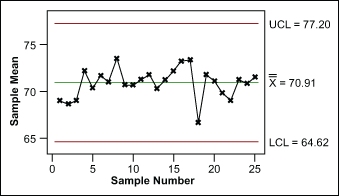Figure 4: Example of Controlled Variation

### Uncontrolled Variation

Uncontrolled variation is characterized by variation that changes over time and is associated with special causes. The outcomes of this process are unpredictable; a customer may be satisfied or unsatisfied given this unpredictability.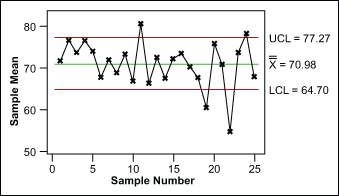Figure 5: Example of Uncontrolled Variation

Please note: process control and process capability are two different things. A process should be stable and in control before process capability is assessed.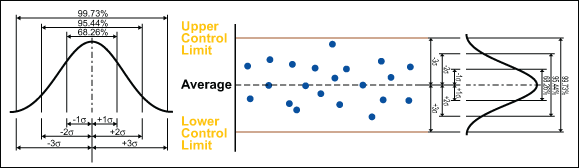Figure 6: Relationship of Control Chart to Normal Curve

### Control Charts for Continuous Data

Individuals and Moving Range Chart

The individuals and moving range (I-MR) chart is one of the most commonly used control charts for continuous data; it is applicable when one data point is collected at each point in time. The I-MR control chart is actually two charts used in tandem (Figure 7). Together they monitor the process average as well as process variation. With x-axes that are time based, the chart shows a history of the process.

The I chart is used to detect trends and shifts in the data, and thus in the process. The individuals chart must have the data time-ordered; that is, the data must be entered in the sequence in which it was generated. If data is not correctly tracked, trends or shifts in the process may not be detected and may be incorrectly attributed to random (common cause) variation. There are advanced control chart analysis techniques that forego the detection of shifts and trends, but before applying these advanced methods, the data should be plotted and analyzed in time sequence.

The MR chart shows short-term variability in a process – an assessment of the stability of process variation. The moving range is the difference between consecutive observations. It is expected that the difference between consecutive points is predictable. Points outside the control limits indicate instability. If there are any out of control points, the special causes must be eliminated.

Once the effect of any out-of-control points is removed from the MR chart, look at the I chart. Be sure to remove the point by correcting the process – not by simply erasing the data point.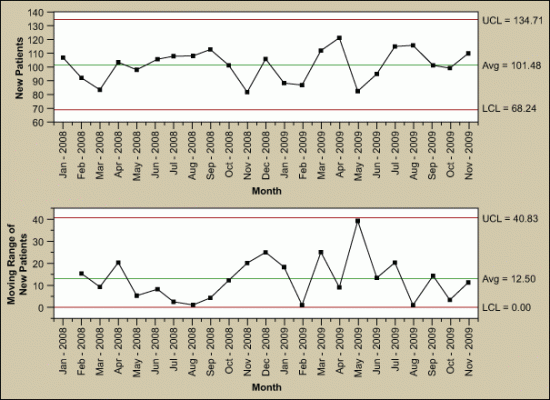Figure 7: Example of Individuals and Moving Range (I-MR) Chart

The I-MR chart is best used when:

1. The natural subgroup size is unknown.
2. The integrity of the data prevents a clear picture of a logical subgroup.
3. The data is scarce (therefore subgrouping is not yet practical).
4. The natural subgroup needing to be assessed is not yet defined.

### Xbar-Range Charts

Another commonly used control chart for continuous data is the Xbar and range (Xbar-R) chart (Figure 8). Like the I-MR chart, it is comprised of two charts used in tandem. The Xbar-R chart is used when you can rationally collect measurements in subgroups of between two and 10 observations. Each subgroup is a snapshot of the process at a given point in time. The chart’s x-axes are time based, so that the chart shows a history of the process. For this reason, it is important that the data is in time-order.

Handpicked Content:   Make Valid Control Chart and Subgroup Assumptions

The Xbar chart is used to evaluate consistency of process averages by plotting the average of each subgroup. It is efficient at detecting relatively large shifts (typically plus or minus 1.5 σ or larger) in the process average.

The R chart, on the other hand, plot the ranges of each subgroup. The R chart is used to evaluate the consistency of process variation. Look at the R chart first; if the R chart is out of control, then the control limits on the Xbar chart are meaningless.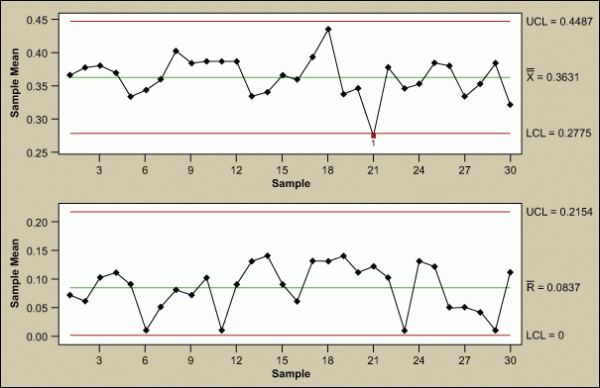Figure 8: Example of Xbar and Range (Xbar-R) Chart

Table 1 shows the formulas for calculating control limits. Many software packages do these calculations without much user effort. (Note: For an I-MR chart, use a sample size, n,  of 2.) Notice that the control limits are a function of the average range (Rbar). This is the technical reason why the R chart needs to be in control before further analysis. If the range is unstable, the control limits will be inflated, which could cause an errant analysis and subsequent work in the wrong area of the process.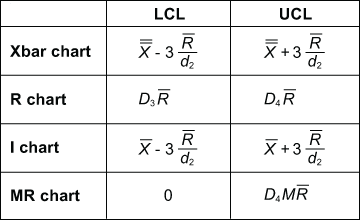Table 1: Control Limit Calculations

 Table 2: Constants for Calculating Control Limits n (Sample Size) d2 D3 D4 2 1.128 – 3.268 3 1.693 – 2.574 4 2.059 – 2.282 5 2.326 – 2.114 6 2.534 – 2.004 7 2.704 0.076 1.924 8 2.847 0.136 1.864 9 2.970 0.184 1.816 10 3.078 0.223 1.777 11 3.173 0.256 1.744 12 3.258 0.283 1.717 13 3.336 0.307 1.693 14 3.407 0.328 1.672 15 3.472 0.347 1.653

Can these constants be calculated? Yes, based on d2, where d2 is a control chart constant that depends on subgroup size.

The I-MR and Xbar-R charts use the relationship of Rbar/d2 as the estimate for standard deviation. For sample sizes less than 10, that estimate is more accurate than the sum of squares estimate. The constant, d2, is dependent on sample size. For this reason most software packages automatically change from Xbar-R to Xbar-S charts around sample sizes of 10. The difference between these two charts is simply the estimate of standard deviation.

### Control Charts for Discrete Data

c-Chart

Used when identifying the total count of defects per unit (c) that occurred during the sampling period, the c-chart allows the practitioner to assign each sample more than one defect. This chart is used when the number of samples of each sampling period is essentially the same.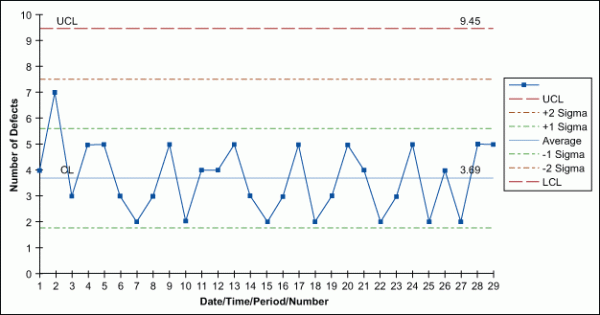Figure 9: Example of c-Chart

u-Chart

Similar to a c-chart, the u-chart is used to track the total count of defects per unit (u) that occur during the sampling period and can track a sample having more than one defect. However, unlike a c-chart, a u-chart is used when the number of samples of each sampling period may vary significantly.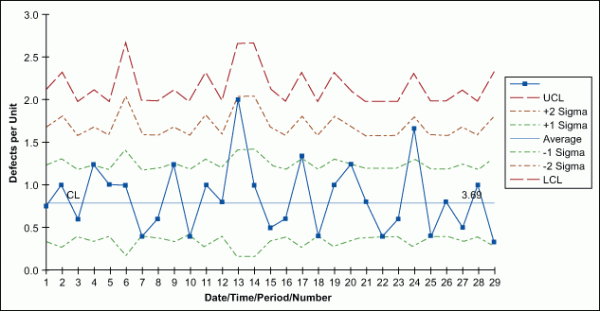Figure 10: Example of u-Chart

np-Chart

Use an np-chart when identifying the total count of defective units (the unit may have one or more defects) with a constant sampling size.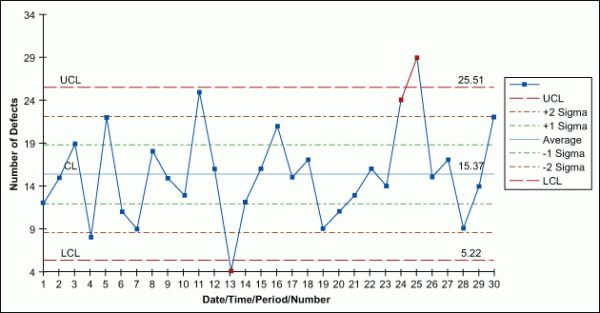Figure 11: Example of np-Chart

p-Chart

Used when each unit can be considered pass or fail – no matter the number of defects – a p-chart shows the number of tracked failures (np) divided by the number of total units (n).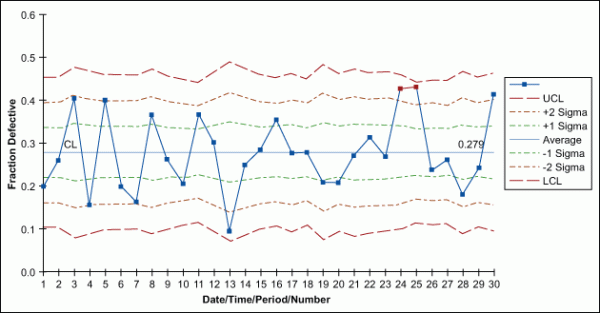Figure 12: Example of p-Chart

Notice that no discrete control charts have corresponding range charts as with the variable charts. The standard deviation is estimated from the parameter itself (p, u or c); therefore, a range is not required.

### How to Select a Control Chart

Although this article describes a plethora of control charts, there are simple questions a practitioner can ask to find the appropriate chart for any given use. Figure 13 walks through these questions and directs the user to the appropriate chart.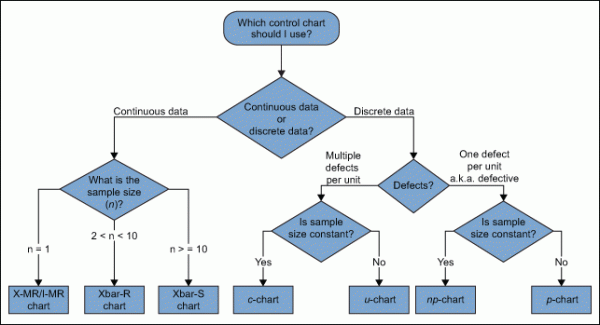Figure 13: How to Select a Control Chart

A number of points may be taken into consideration when identifying the type of control chart to use, such as:

• Variables control charts (those that measure variation on a continuous scale) are more sensitive to change than attribute control charts (those that measure variation on a discrete scale).
• Variables charts are useful for processes such as measuring tool wear.
• Use an individuals chart when few measurements are available (e.g., when they are infrequent or are particularly costly). These charts should be used when the natural subgroup is not yet known.
• A measure of defective units is found with u– and c-charts.
• In a u-chart, the defects within the unit must be independent of one another, such as with component failures on a printed circuit board or the number of defects on a billing statement.
• Use a u-chart for continuous items, such as fabric (e.g., defects per square meter of cloth).
• A c-chart is a useful alternative to a u-chart when there are a lot of possible defects on a unit, but there is only a small chance of any one defect occurring (e.g., flaws in a roll of material).
• When charting proportions, p– and np-charts are useful (e.g., compliance rates or process yields).
Handpicked Content:   A Roadmap for Using Time-weighted Control Charts

### Subgrouping: Control Charts as a Tool for Analysis

Subgrouping is the method for using control charts as an analysis tool. The concept of subgrouping is one of the most important components of the control chart method. The technique organizes data from the process to show the greatest similarity among the data in each subgroup and the greatest difference among the data in different subgroups.

The aim of subgrouping is to include only common causes of variation within subgroups and to have all special causes of variation occur among subgroups. When the within-group and between-group variation is understood, the number of potential variables – that is, the number of potential sources of unacceptable variation – is reduced considerably, and where to expend improvement efforts can more easily be determined.

Within-subgroup Variation

For each subgroup, the within variation is represented by the range.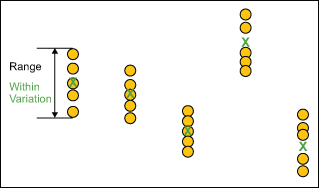Figure 14: Within Subgroup Variation

The R chart displays change in the within subgroup dispersion of the process and answers the question: Is the variation within subgroups consistent? If the range chart is out of control, the system is not stable. It tells you that you need to look for the source of the instability, such as poor measurement repeatability. Analytically it is important because the control limits in the X chart are a function of R-bar. If the range chart is out of control then R-bar is inflated as are the control limit. This could increase the likelihood of calling between subgroup variation within subgroup variation and send you off working on the wrong area.

Within variation is consistent when the R chart – and thus the process it represents – is in control. The R chart must be in control to draw the Xbar chart.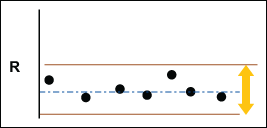Figure 15: Example of R Chart

Between Subgroup Variation

Between-subgroup variation is represented by the difference in subgroup averages.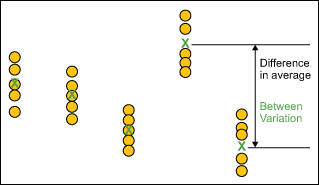Figure 16: Between Subgroup Variation

Xbar Chart, Take Two

The Xbar chart shows any changes in the average value of the process and answers the question: Is the variation between the averages of the subgroups more than the variation within the subgroup?

If the Xbar chart is in control, the variation “between” is lower than the variation “within.” If the Xbar chart is not in control, the variation “between” is greater than the variation “within.”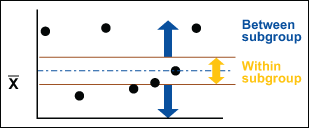Figure 17: Xbar Chart Within Variation

This is close to being a graphical analysis of variance (ANOVA). The between and within analyses provide a helpful graphical representation while also providing the ability to assess stability that ANOVA lacks. Using this analysis along with ANOVA is a powerful combination.

### Conclusion

Knowing which control chart to use in a given situation will assure accurate monitoring of process stability. It will eliminate erroneous results and wasted effort, focusing attention on the true opportunities for meaningful improvement.

### References

1. Quality Council of Indiana. The Certified Six Sigma Black Belt Primer, Second Edition, Quality Council of Indiana, West Terre Haute, Ind., 2012.
2. Tubiak, T.M. and Benbow, Donald W. The Certified Six Sigma Black Belt Handbook, Second Edition, ASQ Quality Press, Milwaukee, Wisc., 2009.
3. Wheeler, Donald J. and Chambers, David S. Understanding Statistical Process Control. SPC Press, Knoxville, Tenn., 1992.
##### Carl Berardinelli

1.Taha Mortaji

Carl, it was great…

2.Peter Tibbetts

Carl,

This was a nice summary of control chart construction. Just wanted to share a couple of my thoughts that I end having to emphasize when introducing SPC.

1) The four points mentioned for the use of the I-mR chart (natural subgroup size is unknown, integrity of the data prevents a clear picture of a logical subgroup, data is scarce, natural subgroup needing to be assessed is not yet defined) do not limit its use to continuous data. Yes, when the conditions for discrete data are present, the discrete charts are preferred. When the conditions are not met, the I-mR will handle the load, so I am a fan of “or I-mR” at the end of each selection path for the discrete charts.
2) I agree the control limits for the Averages (might) be inflated if a Range is out of the control, but if there are still signals on the Average chart, then those signals will be even greater if the limits were not inflated. Even with a Range out of control, the Average chart can and should be plotted with actions to investigate the out of control Ranges.
3) Fortunately Shewhart did the math for us and we can refer to A2 (3/d2) rather than x+3(R-bar/d2).
4) Understanding “Area of Opportunity” for the defect to occur is as important as understanding sample size.

Thanks again. Great article.
Pete

3.Pier Giorgio DELLA ROLE

Hi Carl,
compliments! A great contribution to clarify some basic concepts in Control Charts.

4.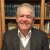5.Kicab

A. Regarding your statements: “Control rules take advantage of the normal curve in which 68.26 percent of all data is within plus or minus one standard deviation from the average, 95.44 percent of all data is within plus or minus two standard deviations from the average, and 99.73 percent of data will be within plus or minus three standard deviations from the average. As such, data should be normally distributed (or transformed) when using control charts, or the chart may signal an unexpectedly high rate of false alarms.”

Just as you were specific in describing several aspects of control charting and distinguishing between the different types, you should be specific about which charts “use” the normal distribution and which don’t.

First, the limits for attribute control charts are based on discrete probability distributions–which, you know, cannot be normal (it is continuous). Thus, no attribute control chart depends on normality.

Second, the range and standard deviations do not follow a normal distribution but the constants are based on the observations coming from a normal distribution. Your statement could apply to the MR-, R-, and S-charts. There is evidence of the robustness (as you say) of these charts.

Third, the Xbar chart easily relies on the central limit theorem without transformation to be approximately normal for many distributions of the observations.

Fourth, even for the I-chart, for many roughly symmetrical or unimodal distributions, the limits are rather robust–as you said.

B. For sample sizes less than 10, that estimate is more accurate than the sum of squares estimate. The d2 factor removes the bias of Rbar conversion as does the c4 factor when using the S-chart, so both are unbiased (if that is what you meant by accurate). On the other hand, R/d2 has more variation than s/c4.
I would use the R-chart over the S-chart regardless of the subgroup size–except possibly if the charts are constructed manually. The reason is that the R-chart is less efficient (less powerful) than the S-chart. In addition, as you indicated, the limits are constructed by converting Rbar into an estimate of the standard deviation by dividing by d2. Why estimate it indirectly–especially if software is doing the calculations?

C. A central line (X) is added as a visual reference for detecting shifts or trends  this is also referred to as the process location.
As with my point (A), this statement depends on the control chart. For the I- and Xbar-charts, the center line is the process location. For all other charts, it is not (or, I am misunderstanding what you mean by process location.)
A better way of understanding the center line on the chart is to recognize that each type of chart monitors a statistic of a subgroup: Xbar monitors averages, R monitors ranges, S monitors standard deviations, c monitors counts, etc. The center line is the average of this statistic across all subgroups.
Now it should be clearer that, for example, the center line of the R-chart cannot be the process locationit is the average range. Similarly, for the S-, MR-, and all the attribute charts.

D. 1. Estimating the standard deviation, ?, of the sample data
2. Multiplying that number by three
3. Adding (3 x ? to the average) for the UCL and subtracting (3 x ? from the average) for the LCL
Mathematically, the calculation of control limits looks like: CL = average ± 3*?hat”

Again, to be clearer, the average in this formula (if applied generically to all control charts) is the average of the statistic that is plotted on the chart. It could be the average of means, the average of ranges, average of counts, etc. The ? that is used on the control limits is not an estimate of the population standard deviation. It is the standard error of the statistic that is plotted. That is, it is the standard deviation of averages in the Xbar-chart, the standard deviation of counts in the c-chart, the standard deviation of standard deviations in the S-chart, and so on.

There is a specific way to get this ?. Because of the lack of clarity in the formula, manual construction of charts is often done incorrectly. This is why it is recommended that you use software.

6.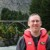Chris Seider

Figure 1 was interesting.

Let’s also not forget to remind people to react to Out of Control indications immediately.

7.Mike Clayton

IMO no one should be using R-bar/d2 these days.
And if they do, think about what the subgrouping assumptions really are.
d2 for sample size of 2 is near 1, while for 9 is near 3.
But what if those samples are correlated, not independent?
Then you limits can be off by 2 or 3 x.
Where is the discussion of correlated subgroup samples and autocorreleated averages for X-bar charts?

If you are ASQ member, check JQT article by Woodall around 2000, with comments from all the gurus, on Issues with SPC. Montgomery deals with many of the issues in his textbook on SPC. But don’t wait to plot the dots and trend the data, just do not assume that the simple textbook methods for setting limits (and rules) are valid for your data source.

8.Wayne G. Fischer, PhD

I think we need to motivate the appropriate use of SPC charts beyond “monitoring” and “analysis.” To me, the use of SPC charts, first and foremost, is to continually *improve* processes – over time. To successfully do that, we must, with high confidence, distinguish between Common Cause and Special Cause variation. We must do *that* because the *actions* we take to deal with each *are different* – and if we confuse the two we make the process’s performance worse.

SPC helps us make good decisions in our continual improvement efforts.

9.Chris Seider

To Wayne G. Fischer.

Isn’t an Out of Control indication by definition a special cause? How would you separate a special cause from the potential common cause variation indicated by the statistical uncertainty? I find your comment confusing and difficult to do practically.

10.Robert Gerst

I disagree with the assertion that;

“Control rules take advantage of the normal curve in which 68.26 percent of all data is within plus or minus one standard deviation from the average, 95.44 percent of all data is within plus or minus two standard deviations from the average, and 99.73 percent of data will be within plus or minus three standard deviations from the average. As such, data should be normally distributed (or transformed) when using control charts, or the chart may signal an unexpectedly high rate of false alarms.”

As Understanding Statistical Process Control, by Wheeler and Chambers is used as a reference by the author, it is worth noting that this same text makes it clear that:

“Myth One: it has been said that the data must be normally distributed before they can be placed on the control chart.”

“Myth Two: It has been said the control charts works because of the central limit theorem.”

The last thing anyone should do when using control charts is testing for normality or transforming the data. These are robust tools for describing real world behavior, not exercises in calculating probabilities. Why remove the very things you are looking for?

11.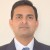To Chris Seider,
Hope the answer lies in broader interpretation of SPC charts that`s beyond control charts. To check special cause presence, Run chart would always be referred. Run chart will indicate special cause existence by way of Trend , osciallation, mixture and cluster (indicated by p value) in the data.Once run chart confirms process stability ,control charts may be leveraged to spot random cause variations and take necessary control measures.

12.To Chris Seider,
Seems i`m not quite right in saying that control charts would just be meant to monitor common cause of variation. While Run chart will definitely highlight process stability (and special cause existence if any), but even control charts can help distinguish between common cause and special cause varaition.There`re rules suggested by “western electric ” and walter shewhart to distinguish between the two causes of variation.Some of them to identify special causes are like-1) any point out of control limits,ii) Nine points in a row in Mean+/- 1sigma or beyond (All on one side.),iii) Six points in a row, all increasing or decreasing,iv) Two out of three points in a row in Mean+/-1 sigma or beyond to name a few and the larger list is anyway there in tools like minitab.Apology for inconvenience.

13.Can the I-MR chart be used to determine an Out-of-Trend of stability test result data during the course of a drug product shelf life?
Kindly appreciate your help on this topic.

•Remarque

No, Stability tracks change in a specific lot over time. Process control tracks how different lots adhere to a target.

Statistics for stability center around multiple regression.

14.Daryn

Hi,
Thanks for a great post!
Could you please provide advice on the following. Every week my team and I complete x number of tasks. Over time we would like to make improvements and increase the average number of completed tasks that we complete.

In most uses, a control chart seems to help to keep a consistent average. Is that true?
What kind of chart could we use to show a gradual increase in the average and also show the upper\lower control limits?

Thanks, Daryn

15.Bill

I’m interested in tracking production data over time, with an 8 hour sample size. This is descrete data. Which control chart is correct?

16.nish

dear sir,

i also learned x bar chart at my university.regarding to this we want to calculate UCL LCL .but i have some question about this.according the formula of using calculate the above figures,the a2 value is constant thing or not? (UCL=x bar-A2(R bar)

17.Samuel Johnson

this is great. It has really helped me understand this concept better.

18.Jeanne Siegel

I am working on P-chart. My LCL is showing as negative but no data falls below zero. How does that effect the mean?

19.Bryan Ostrowski

Really nice summary!

20.D Limit

I have a question about the control limits. Why do we use +/- 3 sigma as UCL/LCL to detect special-cause-variation when we know that the process mean may shift +/- 1,5 sigma over time? The limits in the control chart must be set when the process is in statistical control. However, the amount of data used for this may still be too small in order to account for natural shifts in mean. Why not use 4,5 sigma instead? It’s expensive to stop production.

•Keith Kornafel

Hello D Limit,
I would like to help provide an answer to parts of your question. If I read your question correctly, it illustrates a common point of confusion between Sigma, a measure of dispersion, and Sigma Level, a metric of process capability. They both use the same word–Sigma which can sometimes be confusing. But, Sigma Level and Sigma are NOT EQUIVALENT and many people struggle with this issue.
Sigma Level refers to the number of Sigma, or process standard deviations, between the mean and the closest specification for a process output. Note that when we talk about Sigma Level, this is looking at the process capability to produce within the CUSTOMER SPECIFICATIONS. There is a lot of material out there about the 1.5 shift so I won’t dive into that discussion here – you can read check that out. But the shift is used in the Sigma level to accommodate for process shifts that occur over time. Again, the Sigma level is the measurement of success in achieving a defect-free output which uses the standard deviation and the customers’ specification limit to determine process capability.
Whereas, Sigma in the control charts is about the capability of your PROCESS. You start with the average (or median, mode, and etc.,) which is a measure that represents the standard deviation. You are looking at the process and process capability – you are not looking at the process capability against your customer specifications, so you do not factor in the 1.5 shift on a process chart.
Regards,
Keith Kornafel

21.Sathish Rosario

Dear Carl,

It is a good effort. I learned more about control charts.

I found small variation as follows:

As per the np chart statement: the unit may have one or more defects

As per flow chart “one defect per unit” is noted for np chart.

kindly clarify.

Also some practical examples will provide much more clarity in real use.

Thanks,
Sathish Rosario
[email protected]

22.Dev

Very lucid explanation. Keep writing on such topics.

23.Omkar Naik

If all points in x and R chart lies within UCL and LCL limits ,can all parts be accepted or is there any defetive part present can 6sigma method be used to decide whether or not defective parts are present

24.Prameswari

Hi Carl!
I wanna ask about np control chart for attribute data. There’s a point that lays below the LCL. Why the point is considered as “out of control”? Is not that the smaller defect number the better? Thank you.

Cheers,
Prameswari

25.Kevin

Hi,

This summary helped me a lot but I have still have questions, If I’m working in an assembly with two stations
(A–>B) and I’m having defectives in station A but are still re workable and I can still proceed them to station B. Should I plot those defectives from station A in my p-chart?

26.Pruthvi

They have given just Number of errors and asked to calculate C chart.

27.Omar Abbaas

Thank you for the good article. I have a question about when there is seasonality in the data, the trends are expected to happen and if fixed means and control limits for the entire time period are used, they will indicate false out of control alarms.
What is the best approach to build a control chart for this kind of data, can you please recommend a reference.
Thank you.

28.John Borneman

Very good!! Excellent write up.

29.Kurnia

I found difficulty in interpreting proportion of defect in this kind of data;
I have 10 subgroup, each subgroup has different sampel size. The object that is being inspect is chair and there are 4 observed component per chair. I have been told that control chart used in this case is p chart with proportion of each subgroup is total defective components/(number of chair*4). This is what I’m confused about, what defect proportion is that? Is it the proportion of defective chair or proportion of defective component?

30.Wilfred Hernandez

The I-MR and Xbar-R charts use the relationship of Rbar/d2 as the estimate for standard deviation. What do Xbar-S charts use to estimate standard deviation?. Can you please provide me the equation to calculate UCL and LCL for Xbar-S charts using d constants.

Thank you,

Wil

31.Jigs

Dear Carl,
I am new here, your topics are really informative.I’ve been working in the quality for almost 10 years and want to pursue a career in Quality Engineering. I tried making a control chart but have doubt about it. Example: I have a KEY Diameter of 1.200 ±.001 and want to have a control chart for it. What could be the UCL and LCL?

32.Job Koshy

What is the rationale for selecting this six points for trend and 8 for shift is there any reason behind this tests.

33.Fakhriyor Jamoliddinov

Can you help me with this question? How to solve it?
Company X produces a lot of boxes of Caramel candies and other assorted sweets that are sampled each hour. To set control limits that 95.5% of the sample means, 30 boxes are randomly selected and weighed. The standard deviation of the overall production of boxes iis estimated, through analysis of old records, to be 4 ounces. The average mean of all samples taken is 15 ounces. Calculate control limits for an X – chart.

34.rozina

if all values of x bar are close to central line and none are near 3 sigma limits .in fact, when you draw one sigma limits all the points fall within narrow limits this is called hugging
would such a chart make you suspicious that something was wrong?
why?
what possible explanations occur to you that might account for an x bar chart of this type

35.Jim Rickwa

Very concise and complete explanation. Thanks Carl.

36.Stephen Smith

I am surprised there is no mention of the cumulative sum or exponentially weighted moving average control charts. They are a little more involved than run of the mill control charts but are much more sensitive to change.

37.juanpv19

Referring to the X bar chart. I think it is not quite correct to use UCL = X+ 3*R/d2. The correct way is to use UCL = X+ A2*R. This is because A2 it is equal to 3/(d2* sqr(n)) where n is the size of the subgroup